# Problem: Magic Dates

Date is given in a "dd-mm-yyyy" format, e.g. 17-04-2018. We calculate the weight of that date by taking all of its digits, multiplying each digit with the others after it, and finally summing up all the results obtained. In our case, we have 8 digits: 17032007, so the weight is 1*7 + 1*0 + 1*3 + 1*2 + 1*0 + 1*0 + 1*7 + 7*0 + 7*3 + 7*2 + 7*0 + 7*0 + 7*7 + 0*3 + 0*2 + 0*0 + 0*0 + 0*7 + 3*2 + 3*0 + 3*0 + 3*7 + 2*0 + 2*0 + 2*7 + 0*0 + 0*7 + 0*7 = 144.

Our task is to write a program that finds all the magical dates between two specific years (inclusively) corresponding to given weight. Dates must be printed in ascending order (by date) in the format "dd-mm-yyyy". We will only use the valid dates in the traditional calendar (the leap years have 29 days in February).

## Input Data

Input data should be read from the console. It consists of 3 lines:

• The first line contains an integer: start year.
• The second line contains an integer: end year.
• The third line contains an integer: the search weight for the dates.

Input data will always be valid and will always be in the format described. No need to check.

## Output Data

The result should be printed on the console as consecutive dates in "dd-mm-yyyy" format, sorted by date in ascending order. Each string must be in a separate line. If there are no existing magic dates, print "No".

## Constraints

• The start and final year are integer numbers in the range [1900 … 2100].
• Magic weight is an integer in the range [1 … 1000].
• Allowed program time: 0.25 seconds.
• Allowed memory: 16 MB.

## Sample Input and Output

Input Output Input Output
2007
2007
144
17-03-2007
13-07-2007
31-07-2007
2003
2004
1500
No
Input Output Input Output
2012
2014
80
09-01-2013
17-01-2013
23-03-2013
11-07-2013
01-09-2013
10-09-2013
09-10-2013
17-10-2013
07-11-2013
24-11-2013
14-12-2013
23-11-2014
13-12-2014
31-12-2014
2011
2012
14
01-01-2011
10-01-2011
01-10-2011
10-10-2011

## Hints and Guidelines

We start with the input data. In this case, we have 3 integers that should be read from the console, as this is the only entry and processing of input for the problem.

Having the start and the end year, it is nice to know how we will go through every date, not to worry about how many days there are in the month and whether it is a leap year, and so on.

### Loop through Dates

For looping through the dates, we will take advantage of the functionality that gives us the DateTime class in C#. We will define a start date variable that we can do using the constructor that accepts a year, month, and day. We know the year is the starting year we read from the console and the month and the day must be January and 1st respectively. In C#, the "constructor" of DateTime accepts as first argument the year, as second argument the month and as third argument the day of the month: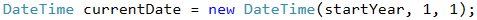Once we have the start date, we want to create a loop that runs until we exceed the final year (or until we pass December 31 in the final year if we compare the full dates), increasing each day by 1 day.

To increase by one day in each rotation, we will use a method of DateTime.AddDays(…), which will add one day to the current date. The method will take care instead of us, to decide where to skip the next month, how many days there is a month and everything around the leap years.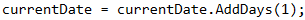Caution: since the .AddDays(…) method returns the "new" date, it is important to assign the result, not just to call the method!

In the end, our loop may look like this: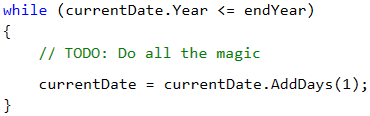Note: we can achieve the same result with a for loop: the initialization of the date goes to the first part of for, the condition is preserved and the step is the increase by 1 day.

### Calculating Date Weight

Each date consists of exactly 8 characters (digits)2 for the day (d1, d2), 2 for the month (d3, d4) and 4 for the year (d5 to d8). This means that we will always have the same calculation every time, and we can benefit from this to define the formula statically (i.e. not to use loops, referring to different numbers from the date, but write the whole formula). To be able to write it, we will need all digits from the date in individual variables to make all the necessary multiplications. By using the division and partition operations on the individual components of the date, using the Day, Month and Year properties, we can retrieve each digit.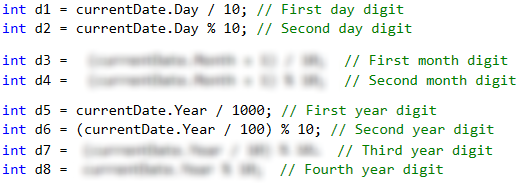Let's also explain one of the more interesting lines here. Let's take the second digit of the year for example (d6). We divide the year by 100, and we take a remainder of 10. What do we do? First, we eliminate the last 2 digits of the year by dividing by 100 (Example: 2018/100 = 20). With the remainder of 10, we take the last digit of the resulting number (20 % 10 = 0) and so we get 0, which is the second digit of 2018.

What remains is to do the calculation that will give us the magical weight of a given date. In order not to write all multiplications as shown in the example, we will simply apply a grouping. What we need to do is multiply each digit with those that follow it. Instead of typing d1 * d2 + d1 * d3 + … + d1 * d8, we can shorten this expression to d1 * (d2 + d3 + … + d8) for grouping when we have multiplication and summing up. Applying the same simplification for the other multiplications, we get the following formula: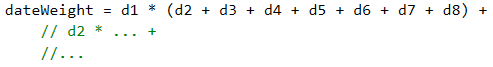### Printing the Output

Once we have the weight calculated of a given date, we need to check and see if it matches the magical weight we want, in order to know if it should be printed or not. Checking can be done using a standard if block, taking care to print the date in the correct format.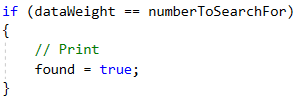To print the dates, we have two options:

• The first way is to use the .ToString(…) method, where we can submit the date format, i.e. whether the days will be printed with a leading zero or not, whether the months will be printed with leading zeros or not, in words or digits, with a short or full name, etc.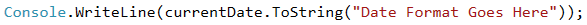• The second option is to take the individual components of the date Day, Month and Year as we did when calculating, and to form the output by formatting string.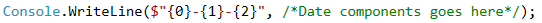Caution: as we go through the dates from the start year to the end one, they will always be arranged in ascending order as per the description.

Finally, if we have not found a date that is eligible, we will have a false value in the found variable and we will be able to print No.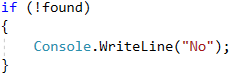## Testing in the Judge System

Test your solution here: https://judge.softuni.org/Contests/Practice/Index/518#1.